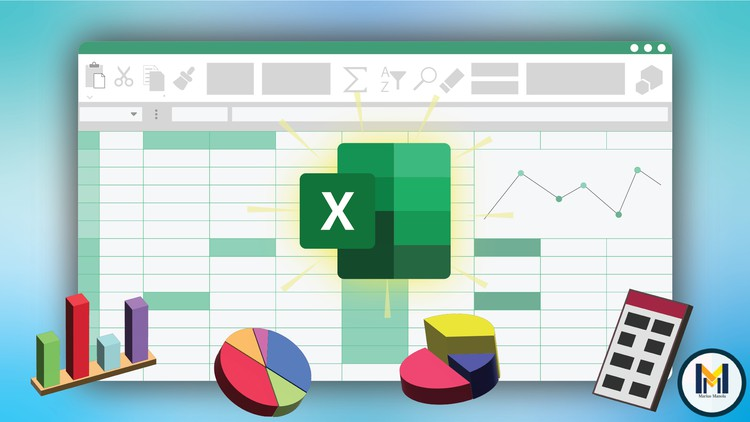# Microsoft Excel – Excel Course from Beginner to AdvancedExcel into Microsoft Excel by taking this ultimate course from beginner to expert in MS EXCEL.

What you will learn

### Learn to use the main Functions

Description

Excel is the most used spreadsheet program in the world, without any doubt. The skills to use Microsoft Excel properly can land you a job these days in no time.

Even though Excel is one of the most used programs in the Microsoft Suite, and there are 1.1 billion Excel users across the globe, most of those people don’t have any idea of how Excel truly works. And those that do know how Excel works, have only some basic knowledge about the program. The magical thing about Excel is that it has been built to make all of your work much easier, and all its tools were made to help you.

Most employees don’t use the special in-built tools that Excel uses, and therefore they have to do everything the old way, which takes an infinitely larger amount of time. With the right amount of knowledge, you can use Excel at work and speed up your work process up to x10.

This course will guide you through the most important tools Excel offers us, and how you can use them to speed up your work process.

————————————————————–

Why learn Excel?

Excel is used for:

1. Data entry
2. Data management
3. Accounting
4. Financial analysis
5. Charting and graphing
6. Programming
7. Time management
9. Financial modeling
10. Customer relationship management (CRM)
11. Almost anything that needs to be organized!

————————————————————–

Here’s a list of the things you’ll know to do after taking this course:

• Know how to use the most basic tools for navigating through the program
• Be able to Input and format your data
• Create tables
• Create charts
• Format/Edit the charts and tables you will create
• Sort data
• Filter data
• Use functions most of the functions
• Much more
English
language

Content

### Introduction into Excel

How to access Microsoft Excel
Introduction into Excel (PART 1)
Introduction into Excel (PART 2)
Introduction into Excel (PART 3)
Introduction into Excel (PART 4)
Introduction into Excel (PART 5)

### Excel Formatting

Introduction
Excel Format Painter
Excel Format Colors
Excel Format Fonts
Excel Format Borders
Excel Number Formats
Excel Regional Format Settings

### Excel Data Analysis

Introduction
Excel Sorting
Excel Filter
Excel Tables
Excel Conditional Formatting
Excel Highlight Cell Rules
Excel Top/Bottom Rules
Excel Above and Below Average Rules
Excel Data Bars
Excel Color Scales
Excel Icon Sets
Excel Manage Rules
Excel Charts (part 1)
Excel Charts (part 2)
Excel Charts (part 3)

Pivot Table
Exercise

### Excel Functions

Introduction
Excel “AND” function
Excel “AVERAGE” function
Excel “AVERAGEIF” function
Excel “AVERAGEIFS” function
Excel “COUNTIF” function
Excel “IF” function
Excel “IFS” function
Excel “LEFT” function
Excel “LOWER” function
Excel “MAX” function
Excel “MEDIAN” function
Excel “MIN” function
Excel “MODE” function
Excel “NPV” function
Excel “OR” function
Excel “RAND” function
Excel “RIGHT” function
Excel “STDEV.P” function
Excel “SUM” function
Excel “TRIM” function
Excel “VLOOKUP” function
Excel “XOR” function
Excel Functions – Summary

### Excel Extras

Introduction
Convert Time into Seconds
Excel Keyboard Shortcuts (part 1)
Excel Keyboard Shortcuts (part 2)